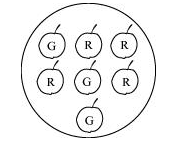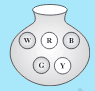## Filters

Sort by :
Clear All
Q

6. Find the probabilities of the events given in Question 2.

2. When a die is thrown, list the outcomes of an event of getting

(i) (a) a prime number

(b) not a prime number.

(ii) (a) a number greater than 5

(b) a number not greater than 5.

Total outcomes = 6 6.(i) (a) prime numbers = 2,3,5 = 3 numbers           probability of prime number                        (b) non prime numbers = 1,4,6 = 3 numbers          probability of non prime number                  (ii) (a) number greater than 5 = 6  = 1 number only     probability of getting number greater than 5                      (b) number not greater than 5 = 1,2,3,4,5 = 5...

5. If you have a spinning wheel with green sectors, blue sector and red sector, what is the probability of getting a green sector? What is the probability of getting a non blue sector?

Total sectors = 5 the probability of getting a green sector                      Non blue sector = 5-1 = 4           probability of getting a non blue sector

4. Numbers 1 to 10 are written on ten separate slips (one number on one slip), kept in a box and mixed well. One slip is chosen from the box without looking into it. What is the probability of .

(i) getting a number ?

(ii) getting a number less than  ?

(iii) getting a number greater than  ?

(iv) getting a 1-digit number?

(i) the probability of getting a number (ii) the numbers less than 6 are: 1,2,3,4,5=5 numbers.     the probability of  getting a number less than   (iii) the numbers greater than 6 are: 7,8,9,10=4 numbers.       the probability of  getting a number greater than      (iv) 1 digit numbers = 1,2,3,4,5,6,7,8,9 = 9 numbers      the probability of  getting a 1 digit  number

3. Find the,

(c) Probability of getting a red apple. (See figure below)(c) Probability of getting a red apple

3. Find the

(b) Probability of getting an ace from a well shuffled deck of 52 playing cards?

(b) Probability of getting an ace from a well shuffled deck of 52 playing cards

3. Find the.

(a) Probability of the pointer stopping on D in (Question 1-(a))?(a) Probability of the pointer stopping on D

2. When a die is thrown, list the outcomes of an event of getting

(i) (a) a prime number (b) not a prime number.

(ii) (a) a number greater than  (b) a number not greater than .

2. When a die is thrown,  the outcomes of an event of getting (i) (a) a prime number  are 2,3,5.     (b) not a prime number are 1,4,6. (ii) (a) a number greater than 5 is 6.       (b) a number not greater than 5 are1,2,3,4,5.

1. List the outcomes you can see in these experiments

(b) Tossing two coins together

(b)The outcomes you can see in the experimentsTossing two coins together are : (i) Head (ii) tail

1. List the outcomes you can see in these experiments.

(a) Spinning a wheel(a) the outcomes you can see in these experiment are:    (i)   A   (ii)  B   (iii)  C   (iv)  D

Suppose you spin the wheel

(iii) Find the probability of not getting a green sector.(iii) the probability of not getting a green sector =  =

Suppose you spin the wheel

(ii) Find the probability of getting a green sector.

.(ii) the probability of getting a green sector = 5/8 = 0.625

Suppose you spin the wheel

1. (i) List the number of outcomes of getting a green sector and not getting a green sector on this wheel (Fig 5.11).(i) the number of outcomes of getting a green sector = 5    the number of outcomes of not getting a green sector = 3

In throwing a die:

• Does the first player have a greater chance of getting a six?

• Would the player who played after him have a lesser chance of getting a six?

• Suppose the second player got a six. Does it mean that the third player would not have a chance of getting a six?

In throwing a die: • No, the first player also have same chance of getting a six. • No, the player who played after him have same chance of getting a six. • Suppose the second player got a six.It does not mean that the third player would not have a chance of getting a six. Third person also have same chance of getting six.

4. You have a bag with five identical balls of different colours and you are to pull out (draw) a ball without looking at it; list the outcomes you would get (Fig 5.10).Fig 5.10

Fig 5.10 The possible outcomes are: (i) W (ii) R (iii) B (iv) G (v) Y

3. When you spin the wheel shown, what are the possible outcomes? (Fig 5.9) List them.

(Outcome here means the sector at which the pointer stops).3. When we spin the wheel shown, the possible outcomes are: (i) A (ii) B (iii) C

2. When a die is thrown, what are the six possible outcomes?

2. When a die is thrown, the six possible outcomes are : (i) 1 (ii) 2 (iii)3 (iv)4 (v)5 (vi)6

1. If you try to start a scooter, what are the possible outcomes?

1.If you try to start a scooter,then there are two possibilities. (i) . The scooter will start. (ii) . The scooter will not start.

5. The number of students in a hostel, speaking different languages is given below. Display the data in a pie chart.The pie chart is as shown below:

4. The adjoining pie chart gives the marks scored in an examination by a student in Hindi, English, Mathematics, Social Science and Science. If the total marks obtained by the students were, answer the following questions.

(iii) Examine whether the sum of the marks obtained in Social Science and Mathematics is more than that in Science and Hindi.

(Hint: Just study the central angles).subject central angle marks obtained mathematics        science      social science      english      hindi      (iii) the sum of the marks obtained in Social Science and Mathematics =       the sum of the marks obtained in Science and Hindi =  Yes, the sum of the marks obtained in Social Science and Mathematics is more than that in Science and Hindi.

4. The adjoining pie chart gives the marks scored in an examination by a student in Hindi, English, Mathematics, Social Science and Science. If the total marks obtained by the students were , answer the following questions.

(ii) How many more marks were obtained by the student in Mathematics than in Hindi?subject central angle marks obtained mathematics        science      social science      english      hindi      (ii) Hence, 30 more marks were obtained by the student in Mathematics than in Hindi.
Exams
Articles
Questions